# JPWO2005020427A1 - Temperature compensated piezoelectric oscillator and electronic device provided with the same - Google Patents

Temperature compensated piezoelectric oscillator and electronic device provided with the same Download PDF

## Info

Publication number
JPWO2005020427A1
JPWO2005020427A1 JP2005513243A JP2005513243A JPWO2005020427A1 JP WO2005020427 A1 JPWO2005020427 A1 JP WO2005020427A1 JP 2005513243 A JP2005513243 A JP 2005513243A JP 2005513243 A JP2005513243 A JP 2005513243A JP WO2005020427 A1 JPWO2005020427 A1 JP WO2005020427A1
Authority
JP
Japan
Prior art keywords
temperature
voltage
piezoelectric oscillator
circuit
compensated piezoelectric
Prior art date
Legal status (The legal status is an assumption and is not a legal conclusion. Google has not performed a legal analysis and makes no representation as to the accuracy of the status listed.)
Granted
Application number
JP2005513243A
Other languages
Japanese (ja)
Inventor

Original Assignee

Priority date (The priority date is an assumption and is not a legal conclusion. Google has not performed a legal analysis and makes no representation as to the accuracy of the date listed.)
Filing date
Publication date
Priority to JP2003297729 priority Critical
Priority to JP2003297729 priority
Application filed by 株式会社村田製作所 filed Critical 株式会社村田製作所
Priority to PCT/JP2004/008338 priority patent/WO2005020427A1/en
Publication of JPWO2005020427A1 publication Critical patent/JPWO2005020427A1/en
Granted legal-status Critical Current

• 230000000875 corresponding Effects 0.000 claims description 10
• 238000006243 chemical reactions Methods 0.000 claims description 4
• 229910052904 quartz Inorganic materials 0.000 claims description 2
• 239000010453 quartz Substances 0.000 claims description 2
• 229910001885 silicon dioxide Inorganic materials 0.000 claims description 2
• 239000003990 capacitor Substances 0.000 description 12
• 238000010586 diagrams Methods 0.000 description 12
• 238000004891 communication Methods 0.000 description 7
• 230000000694 effects Effects 0.000 description 5
• 238000004088 simulation Methods 0.000 description 4
• 230000003321 amplification Effects 0.000 description 3
• 238000003199 nucleic acid amplification method Methods 0.000 description 3
• 230000005540 biological transmission Effects 0.000 description 2
• 238000010295 mobile communication Methods 0.000 description 2
• 230000000051 modifying Effects 0.000 description 2
• 229920000729 poly(L-lysine) polymers Polymers 0.000 description 2
• 239000000919 ceramics Substances 0.000 description 1
• 230000001419 dependent Effects 0.000 description 1
• 230000001629 suppression Effects 0.000 description 1
• 238000010897 surface acoustic wave method Methods 0.000 description 1

## Images

•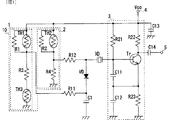•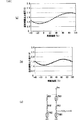•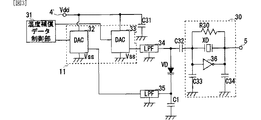•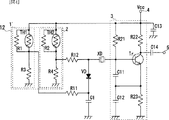•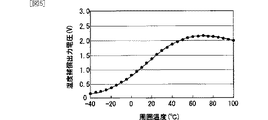••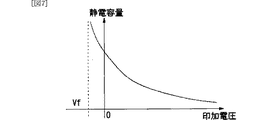•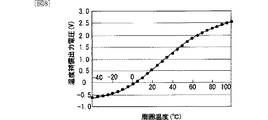•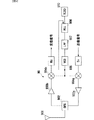## Classifications

• HELECTRICITY
• H03BASIC ELECTRONIC CIRCUITRY
• H03BGENERATION OF OSCILLATIONS, DIRECTLY OR BY FREQUENCY-CHANGING, BY CIRCUITS EMPLOYING ACTIVE ELEMENTS WHICH OPERATE IN A NON-SWITCHING MANNER; GENERATION OF NOISE BY SUCH CIRCUITS
• H03B5/00Generation of oscillations using amplifier with regenerative feedback from output to input
• H03B5/02Details
• H03B5/04Modifications of generator to compensate for variations in physical values, e.g. power supply, load, temperature
• HELECTRICITY
• H03BASIC ELECTRONIC CIRCUITRY
• H03BGENERATION OF OSCILLATIONS, DIRECTLY OR BY FREQUENCY-CHANGING, BY CIRCUITS EMPLOYING ACTIVE ELEMENTS WHICH OPERATE IN A NON-SWITCHING MANNER; GENERATION OF NOISE BY SUCH CIRCUITS
• H03B5/00Generation of oscillations using amplifier with regenerative feedback from output to input
• H03B5/30Generation of oscillations using amplifier with regenerative feedback from output to input with frequency-determining element being electromechanical resonator
• H03B5/32Generation of oscillations using amplifier with regenerative feedback from output to input with frequency-determining element being electromechanical resonator being a piezo-electric resonator
• H03B5/36Generation of oscillations using amplifier with regenerative feedback from output to input with frequency-determining element being electromechanical resonator being a piezo-electric resonator active element in amplifier being semiconductor device
• H03B5/366Generation of oscillations using amplifier with regenerative feedback from output to input with frequency-determining element being electromechanical resonator being a piezo-electric resonator active element in amplifier being semiconductor device and comprising means for varying the frequency by a variable voltage or current
• H03B5/368Generation of oscillations using amplifier with regenerative feedback from output to input with frequency-determining element being electromechanical resonator being a piezo-electric resonator active element in amplifier being semiconductor device and comprising means for varying the frequency by a variable voltage or current the means being voltage variable capacitance diodes
• HELECTRICITY
• H03BASIC ELECTRONIC CIRCUITRY
• H03LAUTOMATIC CONTROL, STARTING, SYNCHRONISATION, OR STABILISATION OF GENERATORS OF ELECTRONIC OSCILLATIONS OR PULSES
• H03L1/00Stabilisation of generator output against variations of physical values, e.g. power supply
• H03L1/02Stabilisation of generator output against variations of physical values, e.g. power supply against variations of temperature only
• H03L1/028Stabilisation of generator output against variations of physical values, e.g. power supply against variations of temperature only of generators comprising piezo-electric resonators

## Abstract

Provided is a temperature compensated piezoelectric oscillator that outputs a high-frequency signal having a constant oscillation frequency even when the power supply voltage is low. The temperature compensated piezoelectric oscillator includes an AT-cut crystal resonator (XD), an amplifier circuit (3) connected to one end of the crystal resonator (XD), and a varactor connected to the other end of the crystal resonator (XD). A diode (VD) and a temperature compensation voltage generation circuit (10) connected to both ends of the varactor diode (VD) via resistors (R11) and (R12) are provided. The temperature compensation voltage generation circuit (10) includes a thermistor (TH1), (TH3) and resistors (R1), (R3), and is connected to the cathode of the varactor diode (VD). And a thermistor (TH2) and resistors (R2) and (R4), and a second voltage generation circuit (2) connected to the anode of the varactor diode (VD).

## Description

The present invention relates to a piezoelectric oscillator, and more particularly to a temperature-compensated piezoelectric oscillator that corrects an oscillation frequency in accordance with an ambient temperature, and an electronic apparatus including the same.

In general, a piezoelectric oscillator includes a piezoelectric element such as a crystal piece that resonates at a predetermined frequency according to an applied voltage, and an amplification circuit that amplifies and outputs a resonance signal from the piezoelectric element. The resonance frequency of such a piezoelectric element such as a crystal piece is temperature-dependent, and the resonance frequency changes according to the temperature of the element even when the same voltage is applied.

As an oscillator for solving this problem, a temperature compensation type including a variable capacitance element such as a varactor diode connected to a piezoelectric element, and a temperature compensation voltage generation circuit that changes a voltage applied to the variable capacitance element according to an ambient temperature. A plurality of piezoelectric oscillators have been proposed (see, for example, Patent Document 1, Patent Document 2, and Patent Document 3).

In such a temperature compensated piezoelectric oscillator, the resonance frequency depends on the combined capacitance of the piezoelectric element and the variable capacitance element. For this reason, if the voltage applied to the variable capacitance element is adjusted, the capacitance of the variable capacitance element changes. As a result, the combined capacitance changes, and the resonance frequency changes. If this amount of change is set so as to cancel the change in the resonance frequency due to the temperature of the piezoelectric element, a temperature-compensated piezoelectric oscillator that outputs a high-frequency signal with a constant resonance frequency without being influenced by the ambient temperature is configured. .
JP 2002-135053 A JP 2002-76773 A JP-A-6-224635

In each conventional temperature-compensated piezoelectric oscillator, an output voltage from a temperature-compensated voltage generation circuit is applied to one end of a variable capacitance element (for example, a varactor diode), and the other end is set to ground or a constant voltage.

By the way, such a temperature-compensated piezoelectric oscillator is mounted on a mobile communication device or the like and used as a reference signal source. However, in recent years, the voltage of the mobile communication device has been lowered, and accordingly, A temperature-compensated piezoelectric oscillator that is a reference signal source is also required to have a low voltage.

A conventional temperature compensation voltage generation circuit is a circuit including a thermistor that is a temperature sensing element as shown in each of the aforementioned patent documents, and an output voltage corresponding to the temperature is obtained by applying a low voltage to the circuit. Thus, voltage is supplied to the variable capacitance element. As a voltage source for supplying a low voltage to the temperature compensated voltage generation circuit, the power supply voltage of the temperature compensated piezoelectric oscillator is usually used for reasons such as circuit simplification.

For this reason, as described above, when the temperature compensated piezoelectric oscillator is lowered in voltage, the voltage supplied to the temperature compensated voltage generation circuit is lowered, and as a result, the output voltage, that is, the voltage supplied to the variable capacitance element. The maximum value of becomes lower. For this reason, the voltage range to be applied to the variable capacitance element is narrowed, and the possible range of capacitance change is narrowed.

On the other hand, the resonance frequency of a piezoelectric element such as a crystal resonator depends on the temperature change, but does not depend on the applied voltage. does not change.

Thereby, in the voltage range generated from the temperature compensation voltage generation circuit, there is a possibility that the temperature compensation of the resonance frequency of the piezoelectric element is not sufficient.

An object of the present invention is to provide a temperature-compensated piezoelectric oscillator that reliably performs temperature compensation even when the power supply voltage is low and outputs a high-frequency signal having a constant resonance frequency, and an electronic device including the same. It is in.

Therefore, the present invention provides a piezoelectric element, an amplifier circuit connected to one end of the piezoelectric element, a variable capacitance element connected to the other end of the piezoelectric element, and a voltage corresponding to temperature to the variable capacitance element. In a temperature-compensated piezoelectric oscillator having a compensation voltage generation means, a first voltage generation that applies to the compensation voltage generation means a first voltage that varies in the opposite direction to the first voltage depending on the ambient temperature at one end of the variable capacitance element. And a second voltage generating means for applying a second voltage that varies according to the ambient temperature to the other end of the variable capacitance element.

In this configuration, the variable capacitance element connected to the piezoelectric element is variable according to the ambient temperature according to the potential difference between the first voltage generated from the first voltage generating means and the second voltage generated from the second voltage generating means. A voltage is applied. For this reason, if the voltage range that can be generated by the first voltage generating means and the voltage range that can be generated by the second voltage generating means are set differently, than if the one end is set to a constant voltage, A voltage that varies with temperature in a wide voltage range is applied to the variable capacitance element. As a result, the capacitance range that the variable capacitance element can take is widened, and changes according to the ambient temperature. As a result, even if the power supply voltage is lowered, the range that the capacitance can take is not narrowed, and the capacitance changes greatly according to the temperature within this range. The resonance frequency of the resonance circuit composed of the piezoelectric element and the variable capacitance element is corrected by making the capacitance amount changing according to the temperature correspond to the change amount of the resonance frequency due to the temperature of the piezoelectric element.

Further, the present invention is characterized in that the first and second voltage generating means each include at least one temperature sensing element and a plurality of resistance elements.

The invention is also characterized in that the temperature sensitive element is a thermistor.

In this configuration, the first and second voltage generating means for applying a voltage to the variable capacitance element are formed by a simple analog circuit network having a thermistor and a resistor.

The present invention further comprises temperature compensation data generating means for detecting the ambient temperature and generating temperature compensation data corresponding to the detected temperature, and the first and second voltage generating means are provided with the digital temperature correction data. It is characterized by comprising DA conversion means for converting the signal into an analog signal.

In this configuration, temperature compensation data corresponding to the detected temperature is stored in advance by the temperature compensation data generating means, and the temperature compensation data is output to each of the first and second voltage generating means according to the detected temperature. The first and second voltage generating means convert the digital temperature compensation data into an analog voltage signal and apply it to the variable capacitance elements. The capacitance of the variable capacitance element changes according to the potential difference between the applied voltage signal from the first voltage generating means and the voltage signal from the second voltage generating means. Here, by making the temperature compensation data correspond to the amount of change in the resonance frequency due to the temperature of the piezoelectric element, the resonance frequency of the resonance circuit composed of the piezoelectric element and the variable capacitance element is corrected appropriately.

In addition, the present invention is characterized in that the piezoelectric element is an AT-cut quartz crystal resonator.

Further, the present invention is characterized in that the variable capacitance element is a variable capacitance diode (varactor diode).

Further, the present invention is characterized in that the electronic device includes the above-described temperature compensated piezoelectric oscillator.

As described above, according to the present invention, the power supply voltage can be reduced by supplying voltages that vary in opposite directions depending on the temperature from different voltage applying means to both ends of the variable capacitance element that affects the oscillation frequency. A temperature-compensated piezoelectric oscillator that reliably compensates the temperature of the oscillation frequency and outputs a high-frequency signal that does not depend on the temperature of the oscillation frequency can be configured even when the voltage is low.

Further, according to the present invention, since the circuit for generating the temperature compensation voltage is formed by a simple analog circuit using only the thermistor and the resistor, the temperature compensated piezoelectric oscillator can be configured with a simple structure. .

Further, according to the present invention, temperature compensation data corresponding to the ambient temperature is stored in advance, the temperature compensation data is input to different DA conversion circuits, converted into voltage signals, and applied to both ends of the variable capacitance element. By doing so, it is possible to configure a temperature compensated piezoelectric oscillator that reliably compensates the temperature of the oscillation frequency and outputs a high-frequency signal that does not depend on the oscillation frequency even when the power supply voltage is low.

In addition, according to the present invention, by providing the temperature compensated piezoelectric oscillator, it is possible to configure an electronic device that operates stably with a low power supply voltage without being affected by the ambient temperature or the operating temperature.

FIG. 1 is an equivalent circuit diagram showing a configuration of a temperature compensated piezoelectric oscillator according to a first embodiment.
FIG. 2 is a graph showing the temperature dependence of the temperature compensated output voltage (potential difference) of the temperature compensated voltage generator circuit of the temperature compensated piezoelectric oscillator shown in FIG. 1, and the temperature compensated voltage generator circuit of the conventional temperature compensated piezoelectric oscillator It is a graph showing the temperature dependence of a temperature compensation output voltage, and an equivalent circuit diagram of a temperature compensation voltage generation circuit of a conventional temperature compensation type piezoelectric oscillator.
FIG. 3 is an equivalent circuit diagram of a temperature compensated piezoelectric oscillator according to a second embodiment.
FIG. 4 is an equivalent circuit diagram of a temperature compensated piezoelectric oscillator according to a third embodiment.
FIG. 5 is a graph showing the temperature dependence of the temperature compensated output voltage (potential difference) of the temperature compensated voltage generating circuit of the temperature compensated piezoelectric oscillator shown in FIG.
FIG. 6 is an equivalent circuit diagram of a temperature compensated piezoelectric oscillator according to a fourth embodiment.
FIG. 7 is a graph showing applied voltage characteristics of capacitance of a varactor diode VD.
FIG. 8 is a graph showing the temperature dependence of the temperature compensated output voltage (potential difference) of the temperature compensated voltage generating circuit of the temperature compensated piezoelectric oscillator shown in FIG.
FIG. 9 is a block diagram of a communication device that is an example of an electronic device.

A temperature compensated piezoelectric oscillator according to a first embodiment of the present invention will be described with reference to FIGS.

FIG. 1 is an equivalent circuit diagram of a temperature compensated piezoelectric oscillator according to the present embodiment.

As shown in FIG. 1, the temperature-compensated piezoelectric oscillator includes an AT-cut crystal resonator (hereinafter simply referred to as “crystal resonator”) XD that is a piezoelectric element, and an amplifier connected to one end of the crystal resonator XD. A circuit 3 and a varactor diode VD which is a variable capacitance element connected to the other end of the crystal resonator XD, and a temperature compensated voltage generator in which two outputs are connected to both ends of the varactor diode VD through resistors R11 and R12, respectively. Circuit 10.

The temperature compensation voltage generation circuit 10 includes a first voltage generation circuit 1 connected to the resistor R11 and a second voltage generation circuit 2 connected to the resistor R12, which include a power supply voltage (Vcc) terminal 4 and a ground. Connected to.

The first voltage generation circuit 1 includes a parallel circuit of a resistor R1 connected to the Vcc terminal 4 and a thermistor TH1 as a temperature sensing element, and a resistor R3 and a thermistor TH3 connected in series to the parallel circuit. One end of TH3 is grounded. Further, the connection point between the resistor R1 and the parallel circuit of the thermistor TH1 and the resistor R3 is connected to the cathode of the varactor diode VD via the resistor R11.

The second voltage generation circuit 2 includes a parallel circuit of a resistor R2 connected to the Vcc terminal 4 and a thermistor TH2, which is a temperature sensitive element, and a resistor R4 connected in series to the parallel circuit. One end is grounded. Further, the connection point between the resistor R2 and the parallel circuit of the thermistor TH2 and the resistor R4 is connected to the anode of the varactor diode VD via the resistor R12.

The varactor diode VD has an anode connected to the crystal resonator XD and a cathode grounded via a high frequency bypass capacitor C1.

The npn transistor Tr of the amplifier circuit 3 has a base connected to the crystal resonator XD, a collector connected to the Vcc terminal 4 via a resistor R22, and an emitter grounded via a resistor R23 and a capacitor C12. . Further, a feedback capacitor C11 is connected between the emitter and base of the transistor Tr, and a bias current supply resistor R21 is connected between the base and the Vcc terminal 4. Further, the collector of the transistor Tr is connected to the output terminal 5 via the capacitor C14. The Vcc terminal 4 is grounded at a high frequency via a capacitor C13. As a result, the transistor Tr has a negative resistance at the resonance frequency of the crystal resonator XD.

The first voltage generation circuit 1 of the temperature compensation voltage generation circuit 10 divides the power supply voltage Vcc by a voltage division ratio of a parallel circuit of the resistor R1 and the thermistor TH1 and a series circuit of the resistor R3 and the thermistor TH3. A voltage signal is applied to the cathode side of the varactor diode VD via the resistor R11. On the other hand, the second voltage generating circuit 2 has a voltage signal obtained by dividing the power supply voltage Vcc by a voltage dividing ratio of the resistor R2 and the parallel circuit of the resistor R2 and the thermistor TH2 and the resistor R4, and the voltage signal of the varactor diode VD. Applied to the anode side.

The varactor diode VD functions as a capacitive element whose capacitance changes according to the potential difference between the voltage from the second voltage generation circuit 2 and the voltage from the first voltage generation circuit 1.

The crystal resonator XD uses an AT-cut crystal resonator, and the resonance frequency changes in a cubic function with respect to the ambient temperature. The crystal resonator XD forms a resonance circuit with its own capacitance, the capacitance of the varactor diode VD, and the capacitance of the capacitor C1, and resonates with the amplifier circuit 3 at a resonance frequency corresponding to the combined capacitance of these elements.

The transistor Tr of the amplifier circuit 3 operates with the power supply voltage Vcc, oscillates together with the resonance circuit, and outputs an oscillation signal to the output terminal 5.

Here, when the ambient temperature changes, the voltage value of the voltage signal output from the first voltage generation circuit 1 and the voltage value of the voltage signal output from the second voltage generation circuit 2 change.

FIG. 2A shows the temperature dependence of the temperature compensated output voltage (potential difference) of the temperature compensated voltage generating circuit of the temperature compensated piezoelectric oscillator shown in FIG. 1, that is, the voltage applied between the cathode and anode of the varactor diode VD. FIG. 2B is a graph showing the temperature dependence of the temperature compensated output voltage of the temperature compensated voltage generating circuit of the conventional temperature compensated piezoelectric oscillator, that is, the voltage applied between the cathode and the anode of the varactor diode VD, FIG. 2C is an equivalent circuit diagram of a temperature compensated voltage generating circuit of a conventional temperature compensated piezoelectric oscillator. 2A, the resistance values of the resistors in FIG. 1 are R1 = 30 kΩ, R2 = 20 kΩ, R3 = 1 kΩ, R4 = 1 kΩ, and the resistance values of each thermistor at 25 ° C. are TH1 = The simulation results are obtained when 2.31 kΩ, TH2 = 46.2 kΩ, TH3 = 462Ω, the B constant of the thermistor is about 3000 to 4000, and Vcc = 3V. FIG. 2B shows the resistance value of each resistor in FIG. 2C as R01 = 30 kΩ, R02 = 10 kΩ, R03 = 10 kΩ, and the resistance value of each thermistor at 25 ° C. is TH1 = 18. This is a simulation result when .5 kΩ, TH2 = 1.24 kΩ, TH3 = 201 kΩ, the B constant of the thermistor is about 3000 to 4000, and Vcc = 3V.

Thus, by using the temperature compensated voltage generation circuit of this embodiment, as shown in FIG. 2, even if the same power supply voltage Vcc is supplied, the range of the output potential difference is compared with the conventional about 0.7V. About 1.2V. That is, even when the power supply voltage Vcc is low, it is possible to suppress the range of the voltage (potential difference) applied to the varactor diode from being reduced. This is because the two output voltages of the temperature compensation voltage generation circuit 10 fluctuate in opposite directions at least in a part of the temperature range.

Since the varactor diode VD functions as a capacitive element having a capacitance according to the above-described potential difference, a wider range of capacitance can be obtained than in the conventional example. In other words, the temperature compensation voltage generation circuit having the above-described configuration suppresses the capacitance range of the varactor diode VD from being narrowed even when the power supply voltage is low.

As a result, the combined capacitance of the resonance circuit composed of the crystal resonator XD, the varactor diode VD, and the capacitor C1 is greatly changed, and the resonance frequency of the resonance circuit is greatly changed.

On the other hand, since the crystal resonator XD originally has temperature dependency, the resonance frequency changes when the ambient temperature changes as described above.

Here, each resistor of the temperature compensation voltage generation circuit is previously set so that the amount of change in the resonance frequency due to the amount of change in the capacitance of the varactor diode XD cancels out the amount of change in the resonance frequency due to the temperature change of the crystal unit XD. By setting the thermistor, the resonance frequency can be prevented from changing even when the power supply voltage is low. That is, it is possible to output a high-frequency signal having a stable oscillation frequency without depending on the ambient temperature.

Next, a temperature compensated piezoelectric oscillator according to a second embodiment will be described with reference to FIG. FIG. 3 is an equivalent circuit diagram of the temperature compensated piezoelectric oscillator according to the present embodiment.

As shown in FIG. 3, the temperature-compensated piezoelectric oscillator includes a crystal resonator XD, an amplifier circuit 30 including the crystal resonator XD, and a varactor that is a variable capacitance element connected to the crystal resonator XD via a capacitor C32. A diode VD and a temperature compensation voltage generation circuit 11 having two outputs connected to both ends of the varactor diode VD via low-pass filters LPF34 and LPF35, respectively.

The temperature compensation voltage generation circuit 11 includes a first DA converter 32 connected to the low-pass filter LPF 35 and a second DA converter 33 connected to the low-pass filter LPF 34, which are connected to the drive voltage (Vdd) terminal 4 ′. . The first and second DA converters 32 and 33 are connected to the temperature compensation data control unit 31 and grounded (Vss).

The varactor diode VD has its anode connected to the crystal resonator XD of the amplifier circuit 30 via the capacitor C32 and to the low-pass filter LPF34, and has its cathode grounded via the high-frequency bypass capacitor C1 and low-pass filter. It is connected to the LPF 35.

In the amplifier circuit 30, a crystal resonator XD, an inverter 36, and a resistor R30 are connected in parallel, and these parallel connection points are grounded via capacitors C33 and C34, respectively. The output side of the amplifier circuit 30 (the output side of the inverter 36) is connected to the output terminal 5. Note that the above-described Vdd and Vss are used as the power source of the IC including the inverter 36.

The temperature compensation data control unit 31 stores temperature compensation data corresponding to the ambient temperature in the memory in advance, and reads the temperature compensation data from the memory based on the temperature detected by the temperature detection unit, and the DA converter 32, DA Output to each of the converters 33. Here, the temperature compensation data is data for determining the voltage (potential difference) applied to both ends of the varactor diode VD based on the temperature dependence of the resonance frequency of the crystal resonator XD of the amplifier circuit 30. The DA converter Data to be output to 32 and data to be output to the DA converter 33 are stored.

When the temperature compensation data control unit 31 outputs temperature compensation data corresponding to the detected temperature to the DA converter 32 and the DA converter 33 of the temperature compensation voltage generation circuit 11, the DA converter 32 and the DA converter 33 output the respective temperature compensation data to D. -A conversion and output as an analog voltage signal. These voltage signals are applied to both ends of the varactor diode VD through LPF 34 and LPF 35, respectively.

The varactor diode VD functions as a capacitive element by changing the capacitance according to the difference (potential difference) between the voltage signal from the DA converter 33 and the voltage signal from the DA converter 32.

The crystal resonator XD of the amplifier circuit 30 uses an AT-cut crystal piece, and the resonance frequency changes in a cubic function with respect to the ambient temperature. Since this resonance frequency is affected by the capacitance of the varactor diode, the change in the resonance frequency due to the ambient temperature can be suppressed by changing the capacitance according to the temperature. That is, the temperature compensation data stored in advance in the temperature compensation data control unit 31 is set so as to suppress the fluctuation of the resonance frequency of the crystal resonator XD by the capacitance of the varactor diode VD according to the detected temperature. Therefore, it is possible to output a high-frequency signal having a constant oscillation frequency without temperature dependency.

In the present embodiment, the LPF 34 and the LPF 35 are inserted on the output side of the DA converter 32 and the DA converter 33, but they may be omitted.

Next, a temperature compensated piezoelectric oscillator according to a third embodiment will be described with reference to FIGS.

FIG. 4 is an equivalent circuit diagram of the temperature compensated piezoelectric oscillator according to the present embodiment.

The temperature compensated piezoelectric oscillator shown in FIG. 4 is obtained by replacing the first voltage generating circuit 1 of the temperature compensated piezoelectric oscillator shown in FIG. 1 with a first voltage generating circuit 1 ′, and the other configurations are the same. . Here, the first voltage generation circuit 1 'is obtained by omitting the thermistor TH3 of the first voltage generation circuit 1 of FIG.

FIG. 5 is a graph showing the temperature dependence of the temperature compensated output voltage (potential difference) of the temperature compensated voltage generating circuit of the temperature compensated piezoelectric oscillator shown in FIG. In FIG. 5, the resistance values of the resistors in FIG. 4 are R1 = 50 kΩ, R2 = 100 kΩ, R3 = 20 kΩ, R4 = 1 kΩ, and the resistance values of each thermistor at 25 ° C. are TH1 = 2.31 kΩ. This is a simulation result when TH2 = 46.2 kΩ, the B constant of the thermistor is about 3000 to 4000, and Vcc = 3V.

As described above, with the circuit configuration shown in FIG. 4, the voltage (potential difference) applied to the varactor diode VD has a curved shape that approximates a cubic function, and almost cancels fluctuations in the resonance frequency of the crystal resonator. Can do.

As a result, a temperature-compensated piezoelectric oscillator that outputs a high-frequency signal at a substantially constant oscillation frequency without depending on the ambient temperature can be formed with a simpler structure.

Next, a temperature compensated piezoelectric oscillator according to a fourth embodiment will be described with reference to FIGS.

FIG. 6 is an equivalent circuit diagram of the temperature compensated piezoelectric oscillator according to the present embodiment.

The temperature compensated piezoelectric oscillator shown in FIG. 6 is obtained by replacing the second voltage generating circuit 2 of the temperature compensated piezoelectric oscillator shown in FIG. 4 with a second voltage generating circuit 2 ′. This is the same as the temperature compensated piezoelectric oscillator shown in FIG. Here, the second voltage generating circuit 2 'connects the resistor R4 to the Vcc terminal 4, connects a parallel circuit of the thermistor TH2 and the resistor R2 to the resistor R4, and grounds one end of the parallel circuit. Circuit. The connection point between the resistor R4 and the parallel circuit is connected to the varactor diode VD via the resistor R12.

With this configuration, the varactor diode VD can be obtained by combining the element values (impedance) of the elements (resistors R1 to R4, thermistors TH1 and TH2) of the temperature compensation voltage generation circuit 13 and the B constant of the thermistor. A negative voltage (a forward bias voltage in the case of a diode) can be applied to.

FIG. 7 is a graph showing applied voltage characteristics of the capacitance of the varactor diode VD. In this figure, the forward direction of the applied voltage represents a negative direction in the diode characteristics. As shown in this figure, the capacitance of the varactor diode VD increases as the applied voltage decreases and becomes a negative voltage. This capacitance continues to increase until reaching the voltage Vf at which current begins to flow through the diode.

FIG. 8 is a graph showing the temperature dependence of the temperature compensated output voltage (potential difference) of the temperature compensated voltage generation circuit of the temperature compensated piezoelectric oscillator shown in FIG. In FIG. 8, the resistance values of the resistors in FIG. 6 are R1 = 50 kΩ, R2 = 20 kΩ, R3 = 20 kΩ, R4 = 20 kΩ, and the resistance values of each thermistor at 25 ° C. are TH1 = 23.1 kΩ. This is a simulation result when TH2 = 37.0 kΩ, the B constant of the thermistor is about 3000 to 4000, and Vcc = 3V.

In this way, with the circuit configuration shown in FIG. 6, the voltage (potential difference) applied to the varactor diode VD becomes a curve that approximates a cubic function, and the voltage (potential difference) applied to the varactor diode VD. The range of For this reason, even if a crystal resonator having high temperature dependence, that is, a large amount of change in the resonance frequency with respect to the ambient temperature, is used, fluctuations in the resonance frequency can be almost canceled out.

As a result, it is possible to form a temperature compensated piezoelectric oscillator that outputs a high-frequency signal at a substantially constant oscillation frequency without depending on the ambient temperature.

In each of the above-described embodiments, the temperature compensated piezoelectric oscillator using the Colpitts type oscillation circuit or the inverter oscillation circuit has been described as shown in the figure. However, the Hartley type, the clamp type, the Peers type or the like is used. Produces the same effect. Further, although an oscillation circuit using a bipolar transistor has been described, a field effect transistor may be used. Further, the same effect can be obtained even with an oscillation circuit using a logic element such as a C-MOS. The same effect can be obtained even if circuit elements such as capacitors and inductors are inserted in the temperature compensated voltage generation circuit shown in each of the above embodiments. In addition, the piezoelectric element is not limited to a crystal resonator, and the same effect can be obtained even with a surface acoustic wave resonator, a ceramic resonator using a bulk resonance, a lithium tantalate resonator, a lithium niobate resonator, or the like. .

Next, an electronic device according to a fifth embodiment will be described with reference to FIG.

FIG. 9 is a block diagram of a communication device which is an example of an electronic device.

As shown in FIG. 9, the communication device 90 includes an antenna 901, a duplexer 902, amplification units 903a and 903b, mixing units 904a and 904b, a voltage controlled oscillator 905, a PLL circuit 906, a low-pass filter 907, The temperature compensation type piezoelectric oscillator 910 according to the present invention, a modulation unit Tx, and a demodulation unit Rx are provided.

The PLL circuit 906 receives the output signal of the voltage controlled oscillator 905, compares the phase of this signal with the frequency-divided signal of the oscillation signal of the temperature compensated piezoelectric oscillator 910, and makes the voltage controlled oscillator 905 have a predetermined frequency. Output the control voltage.

The voltage controlled oscillator 905 receives the control voltage at the control terminal via the low-pass filter 907, and outputs a high-frequency signal corresponding to the control voltage. This high-frequency signal is supplied as a local oscillation signal to each of the mixing units 904a and 904b.

The mixing unit 904a mixes the intermediate frequency signal output from the modulation unit Tx and the local oscillation signal and converts them into a transmission signal. This transmission signal is amplified by the amplifying unit 903a and radiated from the antenna 901 via the duplexer 902.

A reception signal from the antenna 901 is amplified by the amplifying unit 903b via the duplexer 902. The mixing unit 904b mixes the reception signal amplified by the amplification unit 903b and the local oscillation signal from the voltage controlled oscillator 905, and converts the mixed signal into an intermediate frequency signal. This intermediate frequency signal is detected by the demodulator Rx.

As described above, by using the temperature compensated piezoelectric oscillator 910 described in each of the above-described embodiments, a small communication device having excellent communication characteristics can be configured.
In the present embodiment, the electronic device using the temperature compensated piezoelectric oscillator of the present invention has been described using the communication device 90, but the electronic device of the present invention is not limited to the communication device.

## Claims (7)

1. A piezoelectric element; an amplifier circuit connected to one end of the piezoelectric element; a variable capacitance element connected to the other end of the piezoelectric element; and compensation voltage generating means for applying a voltage corresponding to the temperature to the variable capacitance element; In the temperature compensated piezoelectric oscillator with
The compensation voltage generating means includes a first voltage generating means for applying a first voltage that varies according to an ambient temperature to one end of the variable capacitance element; A temperature-compensated piezoelectric oscillator comprising: second voltage generating means for applying a second voltage that varies in direction.
2. 2. The temperature compensated piezoelectric oscillator according to claim 1, wherein each of the first and second voltage generating units includes at least one temperature sensing element and a plurality of resistance elements.
3. The temperature compensated piezoelectric oscillator according to claim 2, wherein the temperature sensitive element is a thermistor.
4. Temperature compensation data generating means for detecting the ambient temperature and generating temperature compensation data according to the detected temperature is provided.
2. The temperature compensated piezoelectric oscillator according to claim 1, wherein the first and second voltage generating means include DA conversion means for converting the temperature-compensated data in a digital format into an analog signal.
5. The temperature-compensated piezoelectric oscillator according to claim 1, wherein the piezoelectric element is an AT-cut quartz crystal resonator.
6. 6. The temperature compensated piezoelectric oscillator according to claim 1, wherein the variable capacitance element is a variable capacitance diode.
7. An electronic apparatus comprising the temperature-compensated piezoelectric oscillator according to claim 1.
JP2005513243A 2003-08-21 2004-06-15 Temperature compensated piezoelectric oscillator and electronic device provided with the same Granted JPWO2005020427A1 (en)

## Priority Applications (3)

Application Number Priority Date Filing Date Title
JP2003297729 2003-08-21
JP2003297729 2003-08-21
PCT/JP2004/008338 WO2005020427A1 (en) 2003-08-21 2004-06-15 Temperature compensated piezoelectric oscillator and electronic apparatus comprising it

## Publications (1)

Publication Number Publication Date
JPWO2005020427A1 true JPWO2005020427A1 (en) 2006-10-19

# Family

## Family Applications (1)

Application Number Title Priority Date Filing Date
JP2005513243A Granted JPWO2005020427A1 (en) 2003-08-21 2004-06-15 Temperature compensated piezoelectric oscillator and electronic device provided with the same

## Country Status (4)

US (1) US7205858B2 (en)
JP (1) JPWO2005020427A1 (en)
CN (1) CN1778034A (en)
WO (1) WO2005020427A1 (en)

## Families Citing this family (13)

* Cited by examiner, † Cited by third party
Publication number Priority date Publication date Assignee Title
JP4949265B2 (en) * 2005-11-07 2012-06-06 シチズンホールディングス株式会社 Temperature compensated oscillator and manufacturing method thereof
JPWO2007135821A1 (en) * 2006-05-19 2009-10-01 株式会社村田製作所 Matching device and antenna matching circuit
US7649426B2 (en) * 2006-09-12 2010-01-19 Cts Corporation Apparatus and method for temperature compensation of crystal oscillators
US8843592B2 (en) * 2006-12-20 2014-09-23 Omx Technology Ab System and method for adaptive information dissemination
US20100033329A1 (en) * 2008-08-08 2010-02-11 Xitel Pty. Ltd. Portable Security Container with Movement Detection System
JP2010103881A (en) * 2008-10-27 2010-05-06 Nippon Dempa Kogyo Co Ltd Crystal oscillator
US8729976B2 (en) * 2010-07-13 2014-05-20 Sand 9, Inc. Methods and apparatus for calibration and temperature compensation of oscillators having mechanical resonators
JP5671939B2 (en) * 2010-10-28 2015-02-18 セイコーエプソン株式会社 Piezoelectric oscillation circuit, constant temperature type piezoelectric oscillator
TWI449323B (en) * 2011-03-29 2014-08-11 Richwave Technology Corp Calibration circuit of a frequency generator and compensation circuit thereof
TWI479798B (en) * 2012-09-04 2015-04-01 Wistron Corp Temperature compensation circuit and electronic device with temperature compensation
US9438249B1 (en) * 2015-09-21 2016-09-06 Qualcomm Incorporated Resonant circuit temperature compensation
US10404209B2 (en) * 2016-09-08 2019-09-03 Silicon Laboratories Inc. Compensating for thermal lag in temperature compensated crystal oscillators
CN106911352A (en) * 2017-01-19 2017-06-30 力同科技股份有限公司 Oscillator signal generative circuit, digital intercom terminal and production method

## Family Cites Families (8)

* Cited by examiner, † Cited by third party
Publication number Priority date Publication date Assignee Title
FR2476934B1 (en) * 1980-02-22 1985-05-17 Electronique Piezo Electrici C
JPS63300609A (en) 1987-05-29 1988-12-07 Kinseki Kk Digital temperature compensated crystal oscillator
JPH0181018U (en) 1987-11-18 1989-05-31
JPH06224635A (en) 1993-01-22 1994-08-12 Ribaa Eretetsuku Kk Temperature compensated crystal oscillator
US6040744A (en) * 1997-07-10 2000-03-21 Citizen Watch Co., Ltd. Temperature-compensated crystal oscillator
JP2002076773A (en) 2000-09-01 2002-03-15 Murata Mfg Co Ltd Temperature compensated oscillator and electronic equipment using the same
JP2002135053A (en) 2000-10-26 2002-05-10 Murata Mfg Co Ltd Piezoelectric oscillator and its manufacturing method as well as electronic equipment using piezoelectric oscillator
JP2004048643A (en) 2002-12-09 2004-02-12 Toyo Commun Equip Co Ltd Temperature compensation piezoelectric oscillator

## Also Published As

Publication number Publication date
CN1778034A (en) 2006-05-24
US7205858B2 (en) 2007-04-17
WO2005020427A1 (en) 2005-03-03
US20060022763A1 (en) 2006-02-02

## Similar Documents

Publication Publication Date Title
US6833769B2 (en) Voltage controlled capacitive elements having a biasing network
US8378755B2 (en) Oscillation circuit
US6094103A (en) Multiple feedback loop ring oscillator and delay cell
US6943637B2 (en) Voltage controlled oscillator circuit for a low power electronic device
DE69433900T2 (en) Temperature compensated quartz oscillator
US6278338B1 (en) Crystal oscillator with peak detector amplitude control
JP2016517219A (en) LNA with linear gain between extended dynamic range
US5883550A (en) Crystal oscillator with a temperature-compensating analog circuit
US5898345A (en) Oscillator circuit with first and second frequency control elements
US6239664B1 (en) Low phase noise, wide tuning range oscillator utilizing a one port saw resonator and method of operation
EP1411630A1 (en) Crystal oscillator emulator
EP1547237B1 (en) Integrated, digitally-controlled crystal oscillator
JP6315164B2 (en) Oscillation circuit, vibration device, electronic device, mobile body, vibration device adjusting method, and sensitivity adjustment circuit
JP2004304762A (en) Voltage control-type oscillator, clock transformer, and electronic apparatus
JP2007300623A (en) Oscillation circuit with embedded power supply circuit
US4571558A (en) Voltage controlled crystal oscillator with reduced oscillations at crystal overtones
JP4596770B2 (en) High quality series resonant oscillator
US7139548B2 (en) Semiconductor integrated circuit device and wireless communication system
TWI388128B (en) Frequency controller for a monolithic clock generator and timing/frequency reference
US8058941B2 (en) Voltage control type temperature compensation piezoelectric oscillator
US6833766B2 (en) Adaptive loop gain control circuit for voltage controlled oscillator
EP1505720A1 (en) Tunable frequency, low phase noise and low thermal drift oscillator
US8289094B2 (en) Voltage controlled oscillator (VCO) circuit with integrated compensation of thermally caused frequency drift
US6909336B1 (en) Discrete-time amplitude control of voltage-controlled oscillator
JPWO2003021765A1 (en) Oscillator and communication equipment

## Legal Events

Date Code Title Description
A131 Notification of reasons for refusal

Free format text: JAPANESE INTERMEDIATE CODE: A131

Effective date: 20070717

A02 Decision of refusal

Free format text: JAPANESE INTERMEDIATE CODE: A02

Effective date: 20071204